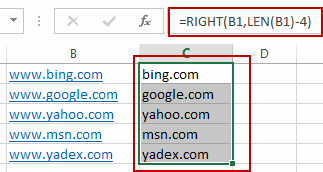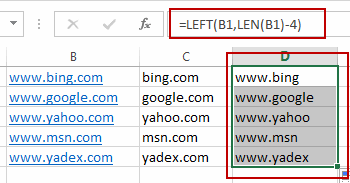# How to Remove Prefix and Suffix in Given Cells in Excel

This post will guide you how to remove suffix form a range of cells in Excel. How do I remove prefix from text string in cells with a formula in Excel.

Assuming that you have a list of data in range B1:B5, you want to remove the prefix “www.”  or suffix “.com” from the text string in cells. Let’s see the following introduction.

## Remove Prefix of Cells

If you want to remove prefix characters from the text string in range B1:B5, you can use a formula based on the RIGHT function and the LEN function. Like this:

`=RIGHT(B1,LEN(B1)-4)`

Type this formula into a blank cell and press Enter key on your keyboard. And then drag the AutoFill Handle down to cells to apply this formula.

Let’s see how this formula works:

Number 4 is the length of your prefix characters. And the Len function returns the length of the cell B1. The RIGHT function will remove the leftmost four characters (prefix) and return the rest characters of the text string.## Remove Suffix of Cells

If you want to remove the suffix characters (.com) from the given range of cells in your worksheet, you can use a formula based on the LEFT function and the Len function. Like this:

`=LEFT(B1,LEN(B1)-4)`

Type this formula into a blank cell and press Enter key on your keyboard. And then drag the AutoFill Handle down to cells to apply this formula.### You might also like:

•Chris Bailey says: